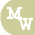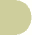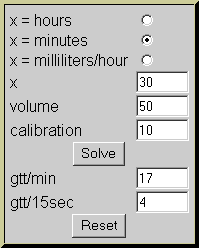calculate IV rate using calibrated tubingnursing calculators search site map

 x = hours x = minutes x = milliliters/hour x volume calibration gtt/min gtt/15sec

Formulas

To calculate rate using minutes

 volume  x  calibration minutes =  gtt/min

To calculate rate using hours

 volume  x  calibration hours  x  60 =  gtt/min

Examples

1)  Give a 50cc IVPB over 30 minutes using IV tubing with a calibration of 10.  How many drops per minute should this IVPB be set for?  See the example below.

select "x = minutes"
x = 30
volume = 50
calibration = 10

Actual formula:

 50 x  10 30 =  16.7 or 17 gtt/min2)  Using IV tubing alone (calibrated at 60), prepare an IV to infuse 1 liter over 8 hours.  How many drops per minute should the IV be set for?  See the example below.

select "x = hours"
x = 8
volume = 1000
calibration = 60

Actual formula:

 1000ml  x  60 8 x 60 =  125 gtt/minNote, when using microdrip tubing (calibration = 60), the drip rate will be the same as mL/hr.  This will save you the time of calculating the drip rate if asked to give the rate in mL/hr.

3)  The doctor orders an IV to infuse at 125cc/hr.  Calculate the flow rate using 10 drop/min IV tubing.

select "x = milliters/hour"
x = 125
calibration = 10

Actual formula:

 125cc x 10 60 = 20.8 or 21 gtt/minNote

You may be wondering why a second answer is displayed in the calculator.  The first answer displays the number of drops per minute.  The second answer displays how many drops fall in 15 seconds.  Sometimes the IV rate is set by counting for 15 seconds instead of a full minute.